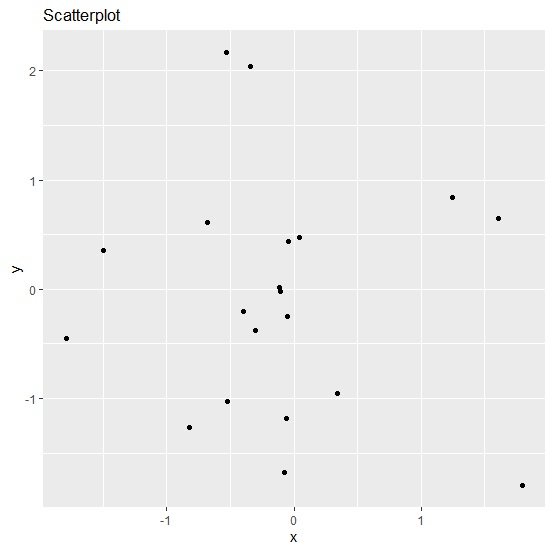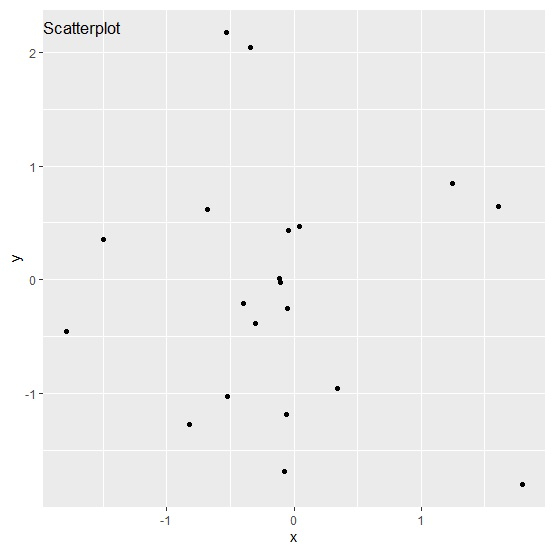# How to put the plot title inside the plot using ggplot2 in R?

Generally, we put the plot title on top side of the plot but we can put it inside the plot as well. Of course, this will change the display of the chart but it will also get attraction of viewers. To do this, we can use the theme function of ggplot2 package where margin argument for plot title will change the position of the title.

Consider the below data frame −

## Example

Live Demo

x<-rnorm(20)
y<-rnorm(20)
df<-data.frame(x,y)
df

## Output

       x           y
1  -0.30662899  -0.37957405
2  -0.82615057  -1.26477881
3  -0.11692952   0.01427444
4  -0.34331938   2.03706444
5  -1.49544241   0.35632086
6  -0.07656434  -1.68049294
7  -0.05300648  -0.24720322
8   1.24363223   0.84503405
9  -0.05653599  -1.18044286
10 -0.04502879   0.43735809
11  0.04637793   0.47158401
12 -0.52382139  -1.02630948
13  1.60555319   0.64673159
14  1.80033105  -1.79870479
15 -0.10558775  -0.02166056
16 -0.68224275   0.61533007
17 -1.79128676  -0.45239927
18  0.34083402  -0.95344404
19 -0.39968860  -0.20690004
20 -0.53267410   2.17089520

Loading ggplot2 package and creating a scatterplot between x and y −

## Example

library(ggplot2)
ggplot(df,aes(x,y))+geom_point()+ggtitle("Scatterplot")

## OutputCreating the scatterplot between x and y with plot title inside the plot area −

## Example

ggplot(df,aes(x,y))+geom_point()+ggtitle("Scatterplot")+theme(plot.title=element_text(margin=margin(t=10,b=-20)))

## Output